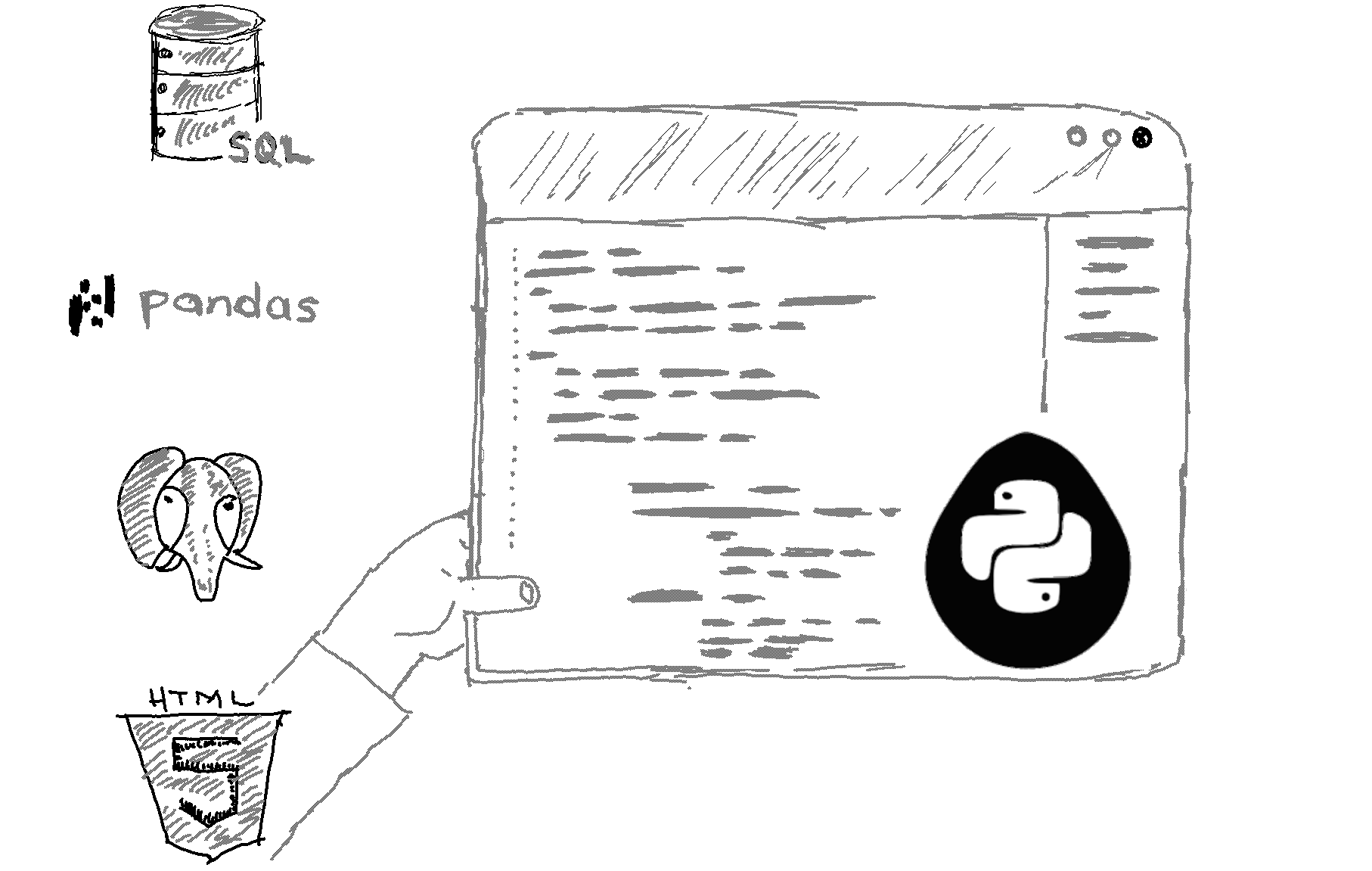# Should I use a for-loop or a list comprehension in Python?

Should you use a for-loop?

`a = range(100000)b = []for i in a:    b.append(i*2)`

Or a list comprehension?

`a = range(100000)b = [i*2 for i in a]`

1. For-loops are more readable and easier to comprehend. It comes more naturally to write a for-loop than it does for a list comprehension.

1. List comprehensions are shorter. A list comprehension can be written in one line whereas a for-loop requires a block of code.
2. List comprehensions are faster:

`import timeitcode_to_test = """a = range(100000)b = []for i in a:    b.append(i*2)"""elapsed_time = timeit.timeit(code_to_test, number=100)/100print(elapsed_time)`

The code above measures the execution time of the for-loop. The output is 0.018875499800778926 seconds.

The code below measures the execution time of a list comprehension that generates the same output as the for-loop above. The output this time in the same machine is  0.007040239260531962 seconnds. So, the list comprehension was 2-3 times faster.

##### Recommended Course

Learn Flask development and learn to build cool apps with our premium Python course on Udemy.